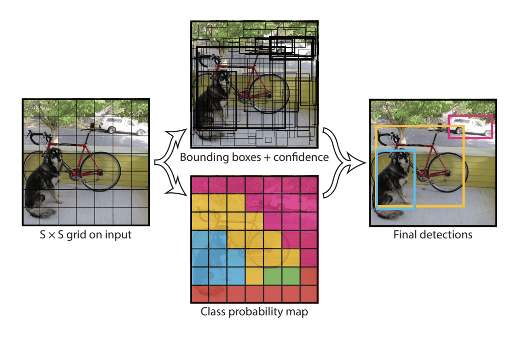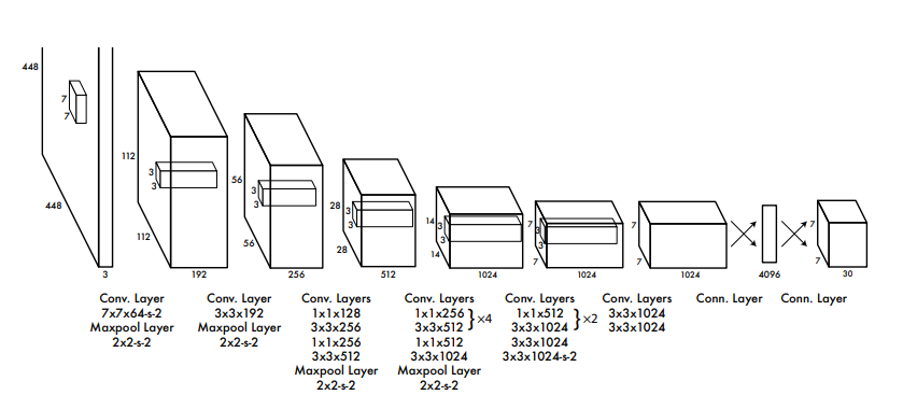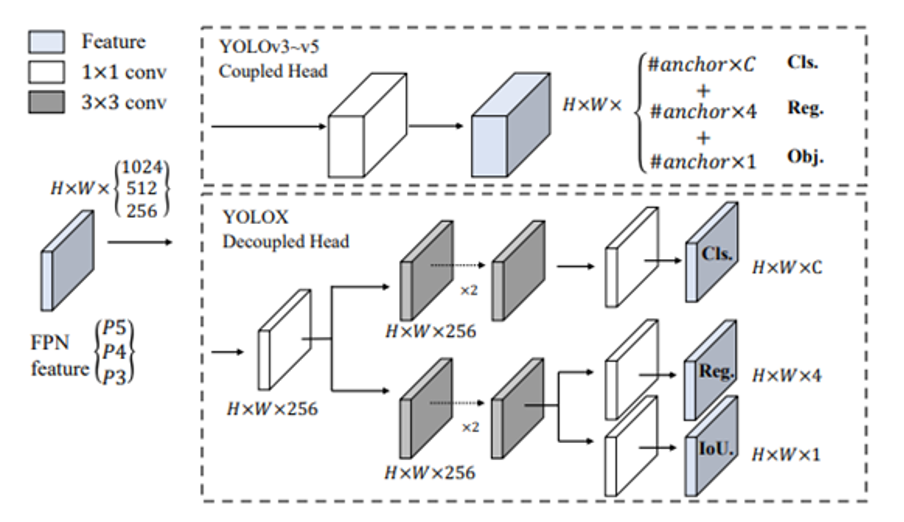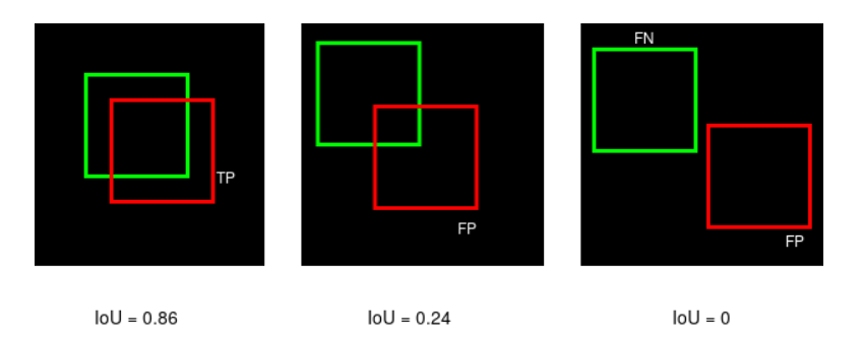Search

# DNN-Based Object Detectors

Updated: May 11

## Introduction, Approaches, Comparisons and Trade-offs

Unlike image classifiers, which simply report on the most important objects within an image, object detectors determine where objects of interest are located, their sizes and class labels within an image. Consequently, object detectors are central to numerous computer vision applications.

In this blog, we provide a technical introduction to deep-neural-network-based object detectors. We explain how these algorithms work, and how they have evolved in recent years, utilizing examples of popular object detectors. We will discuss some of the trade-offs to consider when selecting an object detector for an application, and touch on accuracy measurement. We also discuss performance comparison among the models discussed in this blog.

#### Early Approaches

The seminal work in this regard was reported in the form of technical report at UC Berkeley, in 2014 by Ross Girshick et. al. entitled "Rich feature hierarchies for accurate object detection and semantic segmentation". This is popularly known as “R-CNN” (Regions with CNN features). They approached this problem in a methodical way with having 3 distinct algorithmic stages which is shown in Figure 1 below.Figure 1: R-CNN [“Rich feature hierarchies for accurate object detection and semantic segmentation”]

As seen above, there are three stages:

• Region Proposal: Generate and extract category independent region proposals, using selective search.

• Feature Extractor: Extract feature from each candidate region using a deep CNN

• Classifier. Classify features as one of the known classes using linear SVM classifier model.

Here the main issue was that it produces lots of overlapping bounding boxes and post processing and filtering was needed to produce reliable results. Consequently, it was very slow and require 3 different algorithms to work in tandem. A faster version of this approach was published in 2015 entitled “Fast R-CNN” where authors combined second and third stages into one network with two outputs - one for classification and the other for bounding-box regression.

Shortly afterwards, they published “Faster R-CNN: Towards Real-Time Object Detection with Region Proposal Networks” where region proposal scheme was also implemented using a separate neural network.

## YOLO:

In 2016 a mark departure was proposed by Joseph Redmon et.al. entitled "You Only Look Once: Unified, Real-Time Object Detection" or in short, YOLO. It divides the input image into an S × S grid. If the centre of an object falls into a grid cell, that grid cell is responsible for detecting that object. Each grid cell predicts B bounding boxes, confidence for those boxes, and C class probabilities. The Figure 2 below shows the basic idea.Figure 2: YOLO [You Only Look Once: Unified, Real-Time Object Detection]Figure 3:YOLO Network Architecture

The Figure 3 above shows the network architecture for YOLO detector. The network has 24 convolutional layers followed by 2 fully connected layers. Alternating 1 × 1 layers reduce the features space from preceding layers. The convolutional layers were pretrained on the ImageNet classification task.

The predictions are encoded as an S × S × (B ∗ 5 + C) tensor. YOLO imposes strong spatial constraints on bounding box predictions since each grid cell only predicts two boxes. It can only have one class in a cell. This model struggles with small objects that appear in groups, such as flocks of birds

YOLO-v3 was proposed in 2018 which utilizes multi-resolution approach with three different grid sizes 8x8, 16x16 and 32x32. Objects were learned based on the resolution and anchors. Each resolution has at least 3 anchors to define objects ratios at each cell.

In 2018 YOLO-v4 was proposed, which used Darknet backbone (CSPDarknet53). They utilized advanced training strategies such as mosaic data augmentation, Drop-Block regularization, Class label smoothing, Mish activation, cross-stage partial connections (CSP), Multi-input weighted residual connections. Details of these techniques are beyond the scope of this introduction.

Recently, in 2021 YOLOX was proposed which is anchor free and utilized decoupled output heads for class labels, bounding box regression and IoU, as shown in Figure 4 below.Figure 4: YOLOX

## SSD (Single Shot Detector):

This approach evaluates a small set of default boxes of different aspect ratios (anchor boxes) at each location in several feature maps with different scales (8 × 8 and 4 × 4 in (b) and (c) in Figure 5 below).Figure 5: [SSD: Single Shot MultiBox Detector]

SSD model adds several “feature scaler layers” to the end of a backbone network, which predict the offsets to default boxes of different scales and aspect ratios and their associated confidences. The figure below highlights the differences between YOLO and SSD. For each default box, it predicts both the shape offsets and the confidences for all object categories.Figure 6: SSD vs YOLO

Inference frame rate (speed with batch size 8 using Titan X and cuDNN v4 with Intel Xeon E5-2667v3@3.20GHz.) is 46 fps for SSD of 300x300 pixel images with mAP = 74.3%. Whereas for YOLO (VGG-16) frame rate is 21 fps of 448x448 pixel images with mAP=66.4%. For SSD, output candidate bounding boxes can be very large (~8732) therefore Non-Maxima Suppression (NMS) post-processing needs to be optimized.

## RetinaNet:Figure 7: [Focal Loss for Dense Object Detection]

This technique utilizes Feature Pyramid Network (FPN) backbone on top of feedforward ResNet architecture. They introduced Focal Loss to handle class imbalance in object detection dataset.

RetinaNet with ResNet-101-FPN matches the accuracy of ResNet- 101-FPN Faster R-CNN. The inference time is 172 msec per image on nVidia M40 GPU.

## CenterNet:

In 2019 CenterNet was proposed which had very different approach as compared to other object detection techniques discussed so far. It is trained to produce gaussian blob (heatmaps) at the centre of the object, thereby eliminating the need for anchor boxes as well as NMS post filtering.Figure 8:[Objects as Points]

Object are localized by detecting the local maxima from the heatmaps. Single network predicts the keypoints (local maxima of heatmaps), offset, and size. The network predicts a total of C + 4 outputs at each location.

CenterNet is not the best in terms of accuracy but provides excellent accuracy-speed trade-offs. It can easily be extended to 3D object detection, multi-object tracking and pose estimation etc.

## Evaluation Framework - Accuracy Measurement

True Positive (TP) — Correct detection made by the model. False Positive (FP) — Incorrect detection made by the detector. False Negative (FN) — A Ground-truth missed (not detected) by the object detector.

### Intersection over Union (IoU):

IoU metric in object detection evaluates the degree of overlap between the ground (gt) truth and predicted (pd) bounding boxes. It is calculated as follows:

IoU ranges between 0 and 1, where 0 shows no overlap and 1 means perfect overlap between ground truth and predictions.IoU is used as a threshold (say, α), and using this threshold one can decide if a detection is correct or not. Consequently,

True Positive (TP) is a detection for which IoU(gt,pd)≥ α and False Positive (FP) is a detection for which IoU(gt,pd)< α. False Negative (FN) is a ground-truth missed with gt for which IoU(gt,pd)< αIf we consider IoU threshold, α = 0.5 , then TP, FP and FNs can be identified as shown in the Figure above. If we raise IoU threshold above 0.86, the first instance will be a FP and if we lower IoU threshold below 0.24, the second instance becomes TP.

Precision is the degree of exactness of the model in identifying only relevant objects. It is the ratio of TPs over all detections made by the model.

Recall measures the ability of the model to detect all ground truths—TPs among all ground truths.

A good model is supposed to have high precision and high recall.Raising IoU threshold means that more objects will be missed by the model (more FNs and therefore low recall and high precision). Whereas a low IoU threshold will mean that the model gets more FPs (hence low precision and high recall).

The precision-recall (PR) curve is a plot of precision and recall at varying values of IoU thresholds. As shown in the figure below, the P-R curve is not monotonic, therefore smoothing is applied.Figure 9: Precision-Recall CurveFigure 10: Precision-Recall Curve (smoothed)

#### Average Precision (AP):

AP@α is Area Under the Precision-Recall Curve(AUC-PR) evaluated at α IoU threshold. AP50 and AP75 mean AP calculated at IoU=0.5 and IoU=0.75, respectively.

#### Mean Average Precision (mAP):

There are as many AP values as the number of classes. These AP values are averaged to obtain the metric - mean Average Precision (mAP). Pascal VOC benchmark requires computing AP at 11 points - AP@[0, 0.1, ..., 1.0]. MS COCO describes 12 evaluation metrics shown below.## Comparisons

In this section we summarise and present comparisons which are published in the publicly available research papers.The table above provides comparisons between SSD, YOLO and others on PASCAL VOC 2017 dataset. The tests were done on Titan X GPU and cuDNN v4 with Intel Xeon E5-2667v3@3.20GHz.

Here we see that SSD model is faster with higher mAP but produces lots of boxes and therefore decoding and NMS post filtering can be time consuming.

The table below provides comparison for RetinaNet with others on MS COCO dataset. Here RetinaNet with ResNet-101-FPN and a 600x600 pixel image size (on nVidia M40 GPU) runs at 122 msec per image. It shows that RetinaNet can good very good accuracy with ResNext-101-FPN backbone.The next table below provides comparisons with CenterNet and the previous detectors on COCO dataset. The tests were performed with Intel Core i7-8086K CPU, Titan Xp GPU, Pytorch 0.4.1, CUDA 9.0 and CUDNN 7.1.Here it can be observed that CenterNet gives best tradeoff between FPS and accuracy.

## Choosing An Object Detector

There is no single best detector and choosing one involves looking several factors including application and hardware requirements. In general, highly accurate models are more complex and of larger sizes making them unattractive for embedded or resource constrained devices. On the other hand, smaller models tend to be faster but less accurate. Model size can be reduced, and inference speed can be enhanced using quantization from 32-bit floats to 16-bit floats or 8-bit integers. Obviously, significant boost in speed can be achieved on dedicated hardware such as GPU, NPU etc. at the expense of portability. The table below provides general observations when it comes to deployment.

odel type

<